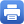Columbia Home
 Home » Programs » Undergraduate Program » Linear AlgebraLinear AlgebraMath UN2010 Linear Algebra: College Bulletin Prerequisite: MATH UN1201 Calculus III, or the equivalent, strongly recommended. Textbook: Otto Bretscher, Linear Algebra with Applications, 5th edition (Publisher link) This course covers matrices, vector spaces, linear transformations, eigenvalues and eigenvectors, canonical forms, and applications. This course provides and introduction to writing mathematical proofs. Students who wish to focus on applications and practical approaches to problem solving should consider taking MATH UN2015 – Linear Algebra & Probability, which is recommended to students majoring in engineering, technology, life sciences, social sciences, and economics. Math majors, joint majors, and math concentrators must take MATH UN2010 – Linear Algebra. Sample syllabus: 1. Linear Equations: 1.1 Introduction to Linear Systems 1.2 Matrices, Vectors, and Gauss-Jordan Elimination 1.3 On the Solutions of Linear Systems; Matrix Algebra 2. Linear Transformations: 2.1 Introduction to Linear Transformations and Their Inverses 2.2 Linear Transformations in Geometry 2.3 Matrix Products 2.4 The Inverse of a Linear Transformation 3. Subspaces of Rn and Their Dimensions: 3.1 Image and Kernel of a Linear Transformation 3.2 Subspace of Rn; Bases and Linear Independence 3.3 The Dimension of a Subspace of Rn 3.4 Coordinates 5. Orthogonality and Least Squares: 5.1 Orthogonal Projections and Orthonormal Bases 5.2 Gram-Schmidt Process and QR Factorization 5.3 Orthogonal Transformations and Orthogonal Matrices 5.4 Least Squares and Data Fitting 6. Determinants: 6.1 Introduction to Determinants 6.2 Properties of the Determinant 6.3 Geometrical Interpretations of the Determinant; Cramer’s Rule 7. Eigenvalues and Eigenvectors: 7.1 Diagonalization 7.2 Finding the Eigenvalues of a Matrix 7.3 Finding the Eigenvectors of a Matrix 8. Symmetric Matrices and Quadratic Forms: 8.1 Symmetric Matrices 8.2 Quadratic Forms 8.3 Singular Values   Help Room Schedule: 406 MathematicsPrint this page